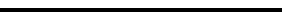# Spectrophotometry

## Basic Principles

### Concepts

A spectrophotometer is employed to measure the amount of light that a sample absorbs. The instrument operates by passing a beam of light through a sample and measuring the intensity of light reaching a detector.

The beam of light consists of a stream of photons, represented by the purple balls in the simulation shown below. When a photon encounters an analyte molecule (the analyte is the molecule being studied), there is a chance the analyte will absorb the photon. This absorption reduces the number of photons in the beam of light, thereby reducing the intensity of the light beam.

You can visualize this process by running the simulation shown below. Click on the Start button to start the simulation and the Stop button to stop the simulation.

The light source is set to emit 10 photons per second. Watch the motion of the photons and observe how some of the photons are absorbed (removed) as the beam of light passes through the cell containing the sample solution. The intensity of the light reaching the detector is less than the intensity emitted by the light source.

 Number of Photons Detected: Elapsed Time: sec Intensity of Detected Light: photons/sec Intensity of Light from the Source: 10.00 photons/sec LightSource Cell Detector

### Experimental Procedure

The following simulation illustrates the procedures for making spectrophotometric measurements.

• First, the intensity of light (I0) passing through a blank is measured. The blank is a solution that is identical to the sample solution except that the blank does not contain the solute that absorbs light. This measurement is necessary, because the cell itself scatters some of the light.

• Second, the intensity of light (I) passing through the sample solution is measured.

• Third, the experimental data is used to calculate two quantities: the transmittance (T) and the absorbance (A).

 T = II0

A = - log10 T

Using the simulation below, perform the following steps:

• Measure the intensity of light passing through the blank.
• Measure the intensity of light passing through the sample.
• Calculate the transmittance.
• Calculate the absorbance.

Note: For each measurement, run the simulation long enough to detect at least 1000 photons. There is substantial random error in the intensity, and the more photons that are counted, the lower the relative uncertainty in the results.

 Blank           Sample Number of Photons Detected: Elapsed Time: sec Intensity of Detected Light: photons/sec LightSource Cell Detector